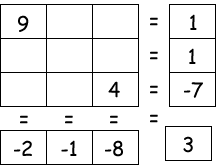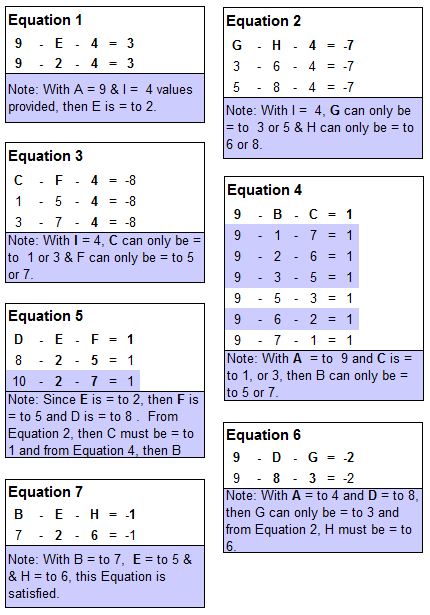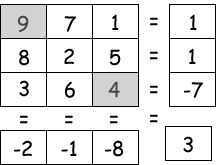Select PagePrintable version

The missing numbers are between 1 & 9. The three numbers in top columns, when subtracted by the lower two numbers, equals the three totals along the bottom, and the three numbers in the left rows, when subtracted by the right two numbers, equals the three totals at the far right. The top left number, when subtracted by the lower two numbers on its diagonal, equals the total in the bottom, far right box. Solve the puzzle by finding all of the missing numbers that satisfy all of the results as shown.### Solution### Solution explanation

Letters (B, C, D, E, F, G and H) have been inserted in the original puzzle example, so that the unknown values can be determined.The puzzle gives us 7 equations with 7 unknown values, as follows:The equations are solved as follows:Giving us the final solution:## Feedback

There are more than one way of doing these puzzles and may well be more than one answer.  Please let me and others know what alternatives you find by commenting below.  We also welcome general comments on the subject and any feedback you’d like to give.   If you have a question that need a response from me or you would like to contact me privately, please use the contact form.

## Get more puzzles!

If you’ve enjoyed doing the puzzles, consider ordering the book; 150+ of the best puzzles in a handy pocket sized format. Click here for full details.